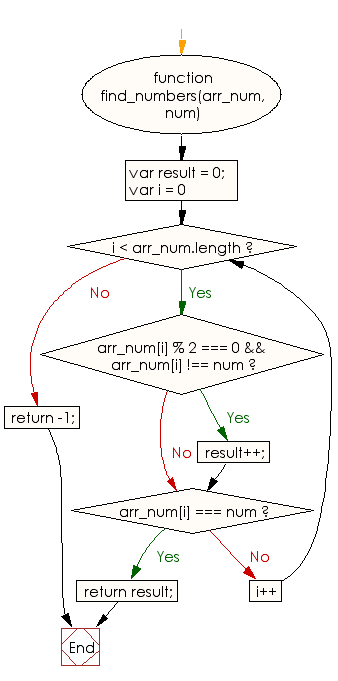# JavaScript: Find the number of even values in sequence before the first occurrence of a given number

## JavaScript Basic: Exercise-110 with Solution

Write a JavaScript program to find the number of even values in sequence before the first occurrence of a given number.

Sample sequence = [1,2,3,4,5,6,7,8]
Given number: 5
Output: 2

Sample Solution:

HTML Code:

``````<!DOCTYPE html>
<html>
<meta charset="utf-8">
<meta name="viewport" content="width=device-width">
<title> Find the number of even values in sequence before the first occurrence of a given number</title>
<body>

</body>
</html>
```
```

JavaScript Code:

``````function find_numbers(arr_num, num) {
var result = 0;
for (var i = 0; i < arr_num.length; i++)
{
if (arr_num[i] % 2 === 0 && arr_num[i] !== num) {
result++;
}
if (arr_num[i] === num)
{
return result;
}
}
return -1;
}

console.log(find_numbers([1,2,3,4,5,6,7,8], 5))
console.log(find_numbers([1,3,5,6,7,8], 6))
``````

Sample Output:

```2
0
```

Flowchart:ES6 Version:

``````function find_numbers(arr_num, num) {
let result = 0;
for (let i = 0; i < arr_num.length; i++)
{
if (arr_num[i] % 2 === 0 && arr_num[i] !== num) {
result++;
}
if (arr_num[i] === num)
{
return result;
}
}
return -1;
}

console.log(find_numbers([1,2,3,4,5,6,7,8], 5))
console.log(find_numbers([1,3,5,6,7,8], 6))
``````

Live Demo:

See the Pen javascript-basic-exercise-110 by w3resource (@w3resource) on CodePen.

What is the difficulty level of this exercise?

Test your Programming skills with w3resource's quiz.

﻿

## JavaScript: Tips of the Day

Checks if a string is an anagram of another string (case-insensitive, ignores spaces, punctuation and special characters)

Example:

```const isAnagram = (str1, str2) => {
const normalize = str =>
str
.toLowerCase()
.replace(/[^a-z0-9]/gi, '')
.split('')
.sort()
.join('');
return normalize(str1) === normalize(str2);
};
console.log(isAnagram('iceman', 'cinema')); // true
```

Output:

```true
```